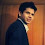# Calculate Hosmer Lemeshow (HL) Test with Excel

Hosmer and Lemeshow Test (HL)

It measures the association between actual events and predicted probability. In other words, it is a measure of how close the predicted probabilities are to the actual rate of events.

In HL test, null hypothesis states that sample of observed events and non-events supports the claim about the predicted events and non-events. In other words, the model fits data well.

Calculation
1. Calculate estimated probability of events
2. Split data into 10 sections based on ascending order of probability
3. Calculate number of actual events and non-events in each section
4. Calculate Predicted Probability = 1 by averaging probability in each section
5. Calculate Predicted Probability = 0 by subtracting Predicted Probability=1 from 1
6. Calculate expected frequency by multiplying number of cases by Predicted Probability = 1
7. Calculate chi-square statistics taking frequency of observed (actual) and predicted events and non-eventsHosmer Lemeshow Test
Rule : If p-value > .05. the model fits data well.
Calculate Hosmer Lemeshow Test with Excel
Related Posts
ShareDeepanshu founded ListenData with a simple objective - Make analytics easy to understand and follow. He has over 10 years of experience in data science. During his tenure, he worked with global clients in various domains like Banking, Insurance, Private Equity, Telecom and HR.

3 Responses to "Calculate Hosmer Lemeshow (HL) Test with Excel"
1.HI Deepanshu I was looking at the attached excel for HL in excel. In the column H, you do an average of all probability (irrespective of dependent variable =0 or 1) and then put that average value in Column I. I was wondering if that is the correct way or we need to filter only those probabilities where dependent variable =1 and then average those probabilities?

1.This is the correct way. Thanks!

2.Thank you very much for posting such a wonderfull tool, it saves a lot of programming and accelerates the work however, I integrated it on my data set and I'm receiving two errors: I have 11 groups instead of 10 and there are negative values. I'm not sure if I missed something during the adaptation or if the problem arises of the wide probabilities range. Would it be possible for you to check my instalation? Thank you very much.

Next → ← Prev
Looks like you are using an ad blocker!

To continue reading you need to turnoff adblocker and refresh the page. We rely on advertising to help fund our site. Please whitelist us if you enjoy our content.